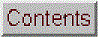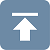[Introduction]  [Signal arithmetic]  [Signals and noise]   [Smoothing]   [Differentiation]  [Peak Sharpening]  [Harmonic analysis]   [Fourier convolution]  [Fourier deconvolution]  [Fourier filter]   [Peak area measurement]  [Linear Least Squares]  [Multicomponent Spectroscopy]  [Iterative Curve Fitting]  [Hyperlinear quantitative absorption spectrophotometry] [Appendix and Case Studies]  [Peak Finding and Measurement]  [iPeak]   [iSignal]  [Peak Fitters]   [iFilter]  [iPower]  [List of downloadable software]  [Interactive tools]# Example of data acquisition and plotting on a Raspberry Pi using Python

This simple example uses the commercially available add-on Sense Hat board in conjunction with a program written in Python. It's applied here to measuring and plotting temperature vs time. The Python code is shown below. (If you don't already have it, install SciPi, NumPy, and Matplotlib into Python according those instructions).

Plug in the Sense Hat board onto the 40 GPIO pins of the Pi, boot it up, install the sense hat software according to instructions, save the Python script to the /home/pi directory as temptime.py, then at the terminal prompt type:

sudo python temptime.py

The results are shown in the animation below. You can see as the temperature of the system warms up and is cooled at time=35 by blowing on it.

I've created modifications of this code to measure and plot atmospheric pressure in millibars, using
pressure = sense.get_pressure(), the percent relative humidity, humidity = sense.get_humidity(), and to calculate and plot the dewpoint from the relative humidity and temperature.  The Sense Hat also comes with a built-in gyroscope, accelerometer, and a magnetometer that can be read in a similar way according to these instructions.

For other examples of Python in such applications, see "
Raspberry Pi for Data Acquisition" and "Think DSP".

Here's the Python code for temperature measurement (adapted from the "decay.py" example included in the Pi's distro):

```import numpy as np
import matplotlib.pyplot as plt
import matplotlib.animation as animation
from sense_hat import SenseHat

sense = SenseHat()

def data_gen(t=0):
cnt = 0
while cnt < 1000:
cnt += 1
t += 0.1
yield t, sense.get_temperature()

def init():
ax.set_ylim(30, 40) # Change as needed to cover the expected temperature range
ax.set_xlim(0, 10)
ax.set_xlabel('Time')
del xdata[:]
del ydata[:]
line.set_data(xdata, ydata)
return line,

fig, ax = plt.subplots()
line, = ax.plot([], [], lw=2)
ax.grid()
xdata, ydata = [], []

def run(data):
# update the data
t, y = data
xdata.append(t)
ydata.append(y)
xmin, xmax = ax.get_xlim()

if t >= xmax:
ax.set_xlim(xmin, 2*xmax)

ax.figure.canvas.draw()
line.set_data(xdata, ydata)

return line,

ani = animation.FuncAnimation(fig, run, data_gen, blit=False, interval=1,
repeat=False, init_func=init)
plt.show()```This page is part of "A Pragmatic Introduction to Signal Processing", created and maintained by Prof. Tom O'Haver , Department of Chemistry and Biochemistry, The University of Maryland at College Park. Comments, suggestions and questions should be directed to Prof. O'Haver at toh@umd.edu. April, 2017.
Unique visits since May 17, 2008: